# Beginning Algebra Tutorial 8

Beginning Algebra
Tutorial 8: Properties of Real NumbersLearning Objectives

After completing this tutorial, you should be able to:
1. Identify and use the addition and multiplication commutative properties.
2. Identify and use the addition and multiplication associative properties.
3. Identify and use the distributive property.
4. Identify and use the addition and multiplication identity properties.
5. Identify and use the addition and multiplication inverse properties.Introduction

It is important to be familiar with the properties in this tutorial.  They lay the foundation that you need to work with equations, functions, and formulas all of which are covered in later tutorials, as well as, your algebra class.   In some cases, it isn't very helpful to rewrite an expression, but in other cases it helps to write an equivalent expression to be able to continue with a  problem and solve it.  An equivalent expression is one that is written differently, but has the same value.  The properties on this page will get you up to speed as to how you can write expressions in equivalent forms.Tutorial

The Commutative Properties of

a + b = b + a       and      ab = ba

The Commutative Property, in general, states that changing the ORDER of  two numbers either being added or multiplied, does NOT change the value of it.

The two sides are called equivalent expressions because they look different but have the same value.Example 1:  Use the commutative property to write an equivalent expression to 2.5x + 3y.

Using the commutative property of addition (where changing the order of a sum does not change the value of it) we get

2.5x + 3y = 3y + 2.5x.Example 2:  Use the commutative property to write an equivalent expression to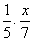.

Using the commutative property of multiplication (where changing the order of a product does not change the value of it), we get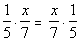The Associative Properties of

a + (b + c) = (a + b) + c     and    a(bc) = (ab)c

The Associative property, in general, states that changing the GROUPING of numbers that are either being added or multiplied does NOT change the value of it.  Again, the two sides are equivalent to each other.

At this point it is good to remind you that both the commutative and associative properties do NOT work for subtraction or division.Example 3:   Use the associative property to write an equivalent expression to   (a + 5b) + 2c.

Using the associative property of addition (where changing the grouping of a sum does not change the value of it) we get

(a + 5b) + 2c = a + (5b + 2c).Example 4:   Use the associative property to write an equivalent expression  to  (1.5x)y.

Using the associative property of multiplication (where changing the grouping of a product does not change the value of it) we get

(1.5x)y = 1.5(xy)

Distributive Properties

a(b + c) = ab + ac
or
(b + c)a = ba + ca

In other words, when you have a term being multiplied times two or more terms that are being added (or subtracted) in a ( ), multiply the outside term times EVERY term on the inside.

Remember terms are separated by + and -.

This idea can be extended to more than two terms in the ( ).Example 5:  Use the distributive property to write 2(x - y) without parenthesis.

Multiplying every term on the inside of the ( ) by 2 we get: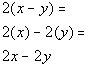*Distribute 2 to EVERY term inside (  )Example 6:  Use the distributive property to write - (5x + 7) without parenthesis.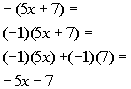*A - outside a ( ) is the same as times (-1)
*Distribute the (-1) to EVERY term inside ( )
*Multiply

Basically, when you have a negative sign in front of a ( ), like this example, you can think of it as taking a -1 times the ( ).  What you end up doing in the end is taking the opposite of every term in the ( ).Example 7:  Use the distributive property to find the product
3(2a + 3b + 4c).

As mentioned above, you can extend the distributive property to as many terms as are inside the ( ).  The basic idea is that you multiply the outside term times EVERY term on the inside.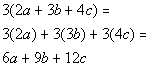*Distribute the 3 to EVERY term
*Multiply

Identity Properties

a + 0 = 0 + a = a

In other words, when you add 0 to any number, you end up with that number as a result.

Multiplication

Multiplication identity is 1

a(1) = 1(a) = a

And when you multiply any number by 1, you wind up with that number as your answer.

The Inverse Properties

For each real number a, there is a unique real number,
denoted -a,
such that

a + (-a) = 0.

In other words, when you add a number to its additive inverse, the result is 0. Other terms that are synonymous with additive inverse are negative and opposite.

Multiplicative Inverse
(or reciprocal)

For each real number a, except 0, there is a unique real number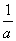such that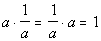In other words, when you multiply a number by its multiplicative inverse the result is 1.  A more common term used to indicate a  multiplicative inverse is the reciprocal.  A multiplicative inverse or reciprocal of a real number a (except 0) is found by "flipping" a upside down.  The numerator of a becomes the denominator of the reciprocal of a and the denominator of a becomes the numerator of the reciprocal of a.

These two inverses will come in big time handy when you go to solve equations later on.  Keep them in your memory bank until that time.Example 8:   Write the opposite (or additive inverse) and reciprocal (or multiplicative inverse) of -3.

The opposite of -3 is 3, since -3 + 3 = 0.

The reciprocal of -3 is -1/3, since -3(-1/3) = 1.

When you take the reciprocal, the sign of the original number stays intact.  Remember that you need a number that when you multiply times the given number you get 1.  If you change the sign when you take the reciprocal, you would get a -1, instead of 1, and that is a no no.Example 9:  Write the opposite (or additive inverse) and reciprocal (or multiplicative inverse) of 1/5.

The opposite of 1/5 is -1/5, since 1/5 + (-1/5) = 0.

The reciprocal of 1/5 is 5, since 5(1/5) = 1.Practice Problems

These are practice problems to help bring you to the next level.  It will allow you to check and see if you have an understanding of these types of problems. Math works just like anything else, if you want to get good at it, then you need to practice it.  Even the best athletes and musicians had help along the way and lots of practice, practice, practice, to get good at their sport or instrument.  In fact there is no such thing as too much practice.

To get the most out of these, you should work the problem out on your own and then check your answer by clicking on the link for the answer/discussion for that  problem.  At the link you will find the answer as well as any steps that went into finding that answer.Practice Problems 1a - 1b: Use a commutative property to write an equivalent expression.

1b.  .1 + 3xPractice Problems 2a - 2b: Use an associative property to write an equivalent expression.

2a. (a + b) + 1.5

2b.  5(xy)Practice Problems 3a - 3b: Use the distributive property to find the product.

3a.  -2(x - 5)
3b.  7(5a + 4b + 3c)Practice Problems 4a - 4b: Write the opposite (additive inverse) and the reciprocal (multiplicative inverse) of each number.

4a.  -7

4b.  3/5Need Extra Help on these Topics?

The following are webpages that can assist you in the topics that were covered on this page:

http://www.mathleague.com/help/wholenumbers/wholenumbers.htm#commutativeproperty
This webpage helps with the commutative property.

http://www.mathleague.com/help/wholenumbers/wholenumbers.htm#associativeproperty
This webpage helps with the associative property.

http://www.mathleague.com/help/wholenumbers/wholenumbers.htm#distributiveproperty
This webpage helps with the distributive property.

This webpage goes over the commutative, associative, and distributive properties.

Go to Get Help Outside the Classroom found in Tutorial 1: How to Succeed in a Math Class for some more suggestions.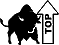Last revised on July 24, 2011 by Kim Seward.